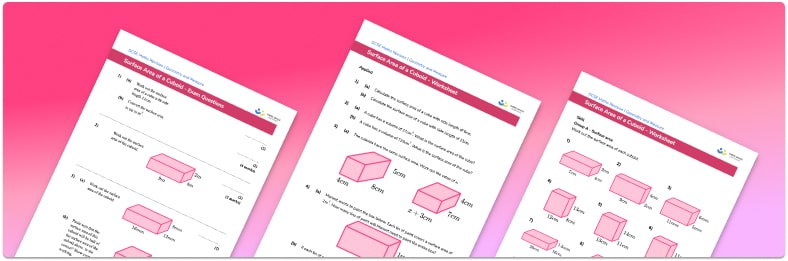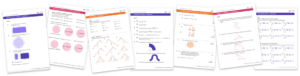# Surface Area Of A Cuboid Worksheet• Section 1 of the surface area of a cuboid worksheet contains 27 skills-based surface area of a cuboid questions, in 3 groups to support differentiation
• Section 2 contains 4 applied surface area of a cuboid questions with a mix of worded problems and deeper problem solving questions
• Section 3 contains 3 foundation and higher level GCSE exam style surface area of a cuboid questions
• Answers and a mark scheme for all surface area of a cuboid questions are provided
• Questions follow variation theory with plenty of opportunities for students to work independently at their own level
• All questions created by fully qualified expert secondary maths teachers

Suitable for GCSE maths revision for AQA, OCR and Edexcel exam boards

• This field is for validation purposes and should be left unchanged.

You can unsubscribe at any time (each email we send will contain an easy way to unsubscribe). To find out more about how we use your data, see our privacy policy.

### Surface area of a cuboid at a glance

Surface area is a measure of the total area of all of the faces of a 3D shape. To find the surface area of a cube or a cuboid, we find the area of the 6 square or rectangular faces and add them to give the total surface area of the cube/cuboid. Remember, to find the area of a rectangle, we multiply the two side lengths.

The total surface area of a cube is the sum of the area of each face, which is a square. This means that if the side length of each face is x , the surface area of a cube is 6x^2 .

The opposing faces of any cube/cuboid are congruent.

For example, calculate the surface area of the rectangular prism where w=5cm, h=2cm, and   d=3cm .

The top and the bottom faces of a cuboid are congruent.

The base = w times d = 5 times 3 = 15cm^2 .

The left and the right faces of a cuboid are congruent.

The left = h times d = 2 times 3 = 6cm^2 .

The front and the back faces of a cuboid are congruent.

The front = w times h = 5 times 2 = 10cm^2 .

The surface area of this cuboid is therefore 15 + 15 + 6 + 6 + 10 + 10 = 62cm^2 .

Surface area is measured in square units, such as centimetres squared or or millimetres squared.

Looking forward, students can then progress to additional surface area worksheets and other geometry and measure worksheets, for example a simplifying expressions worksheet or simultaneous equations worksheet.For more teaching and learning support on Geometry our GCSE maths lessons provide step by step support for all GCSE maths concepts.

## Do you have KS4 students who need more focused attention to succeed at GCSE?There will be students in your class who require individual attention to help them succeed in their maths GCSEs. In a class of 30, it’s not always easy to provide.

Help your students feel confident with exam-style questions and the strategies they’ll need to answer them correctly with our dedicated GCSE maths revision programme.

Lessons are selected to provide support where each student needs it most, and specially-trained GCSE maths tutors adapt the pitch and pace of each lesson. This ensures a personalised revision programme that raises grades and boosts confidence.

Find out more